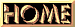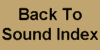CHAPTER III MOTORS AND CONVERTING EQUIPMENT (A) GENERAL THEORY 21. Generation of Electricity by Rotating Machinery.- It was explained in the previous chapter that when a current is passed through a wire, a magnetic field is set up around it, and that a voltage is generated across a loop of wire placed in a magnetic field if the number of magnetic lines of force included within the loop are changed. The magnitude of the voltage generated depends upon the rate at which the number of lines of force through the loop are changed, i.e., if a change of ten lines of force per second produces a certain voltage, a change of twice ten, or twenty, lines per second produces twice the voltage. The total number of line through the loop at the time of the change has no effect upon the voltage generated Specifically stated, this means that the same voltage is produced in a coil when the number of lines is changed from 100 to 120 in one second, as when the number of lines is changed from 1,000 to 1,020 in one second, because the rate of change of the number of lines through the loop is the same (20 lines per second) in each case. The direction of the generated voltage depends upon the direction of the line: of force through the loop, and upon whether the number of lines of force through the loop are increasing or decreasing. The process of following through the different changes of position of a rotating loop so as to determine the nature of the generated voltage in it, is complicated and not easily understood. The generation of a voltage in a loop can be more readily understood if the sides of the loop are thought of as cutting the lines of force. When so considered the voltage generated is proportional to the number of lines of force cut per second, and the direction of the voltage generated depend: upon the direction in which the sides of the loop cut the lines of force. Figure 11 shows a magnetic field in which there is a loop of wire. The magnetic lines of force flow from the north pole to the south pole in parallel lines across the air gap between the poles. In (a) a loop of wire is shown in a position such that one side of the loop is cutting the lines of force in one direction, and the other side of the loop is cutting the lines in the other direction. The direction in which the loop is turning is shown by the arrow at the crank. Under this condition a voltage is generated in side "A" so as to cause current to flow in the direction shown by the arrow at that side of the loop. Side "B" of the loop cuts the lines of force in the opposite direction, therefore the voltage generated in side "B" causes a current to flow in the opposite direction, as shown by the arrow a that side of the loop. The voltage generated in the sides of the loop causes a current to flow into one side and out of the other. In order for a current actually to 1

 Chapter Three Pages                           

 Chapter©1930 RCA Photophone, Inc
HTML Transcription & Graphic Reproductions ©2000 The American WideScreen Museum
All Rights Reserved